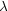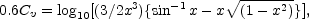### SUMMARY OF OBSERVATIONAL RESULTS

(a) Laws of Red-Shifts

(Stationary Universe)             d/= k r

(Expanding    '' )                                   = kr + l r2 + ...

(k = 5.37 × 10-10; l = 2.54 × 10-19; r = distance)

(b) Laws of Nebular Distribution

(Stationary Universe)                             log10 Nm = 0.6 mc + Cs

(Expanding ,, )                                                           = 0.6(mc - d/+ Cv) + Ce

Cs = - 4.437; Ce = - 4.391; Nm = number of nebulae over entire sky, brighter than mc; mc = apparent magnitude corrected for local obscuration and for energy effects of red-shifts; d/= mean red-shift at distance corresponding to mc. The term Cv is represented by the equationin which x = r / R0 where R0 is the present radius of spatial curvature, and r is the distance corresponding to apparent magnitude (mc - d/) .

(c) Derived Constants in an Expanding Universe

Present Radius of Spatial Curvature       = R0 = 4.7 × 108 (light-years).

Cosmological Constant         = 4.5 × 10-18 (years)-2.

Present Mean Density           = 6 × 10-27 gm./cm3.

The table neatly summarizes the explorations in the observable region of space, the immense region within which reliable data can be gathered with the largest telescope in operation. The results are a definite step in the observational approach to cosmology. The field is entered, the problems formulated, and the solutions restricted to a fairly narrow range. But the essential clue, the interpretation of red-shifts, must still be unravelled. The former sense of certainty has faded and the clue stands forth as a problem for investigation.

Larger telescopes may resolve the question, or theory may be revised to account for the new data. But with regard to relativistic cosmology in its present form, and the observations now available, the conclusion can be stated quite simply. Two pictures of the universe are sharply drawn. Observations, at the moment, seem to favour one picture, but they do not rule out the other. We seem to face, as once before in the days of Copernicus, a choice between a small, finite universe, and a universe indefinitely large plus a new principle of nature.